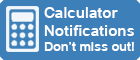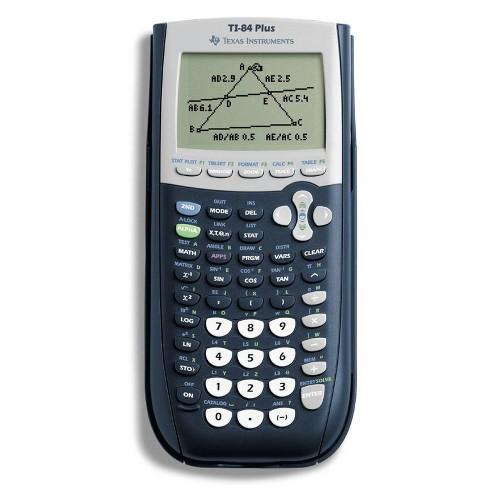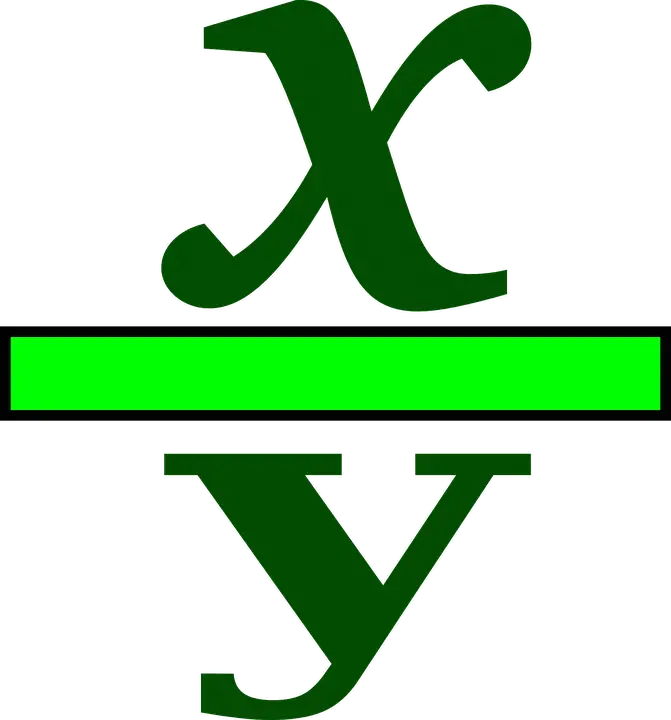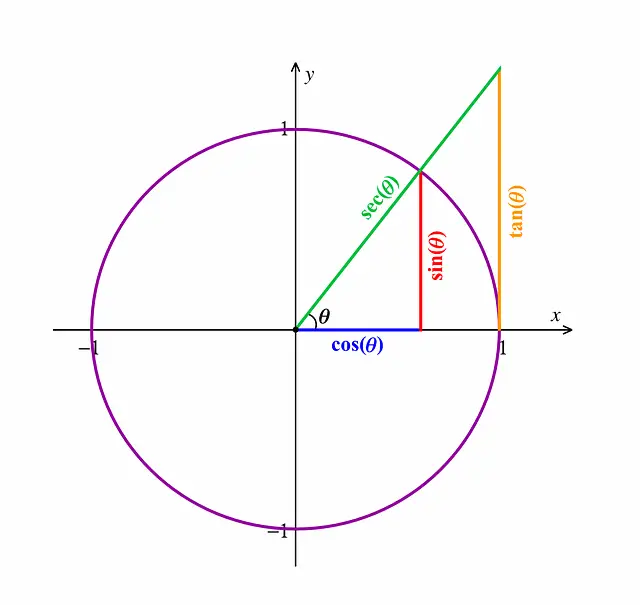Calculator Library
Free Online CalculatorsHome » 87 Calculators » Blog » How To Do Fractions On A Calculator?

Huge Domain Name Sale | Free Domains
Domain names on sale at crazy prices. Register a new domain for FREE when you buy another service.

## HOW TO DO FRACTIONS ON A CALCULATOR?If you're looking for how to do fractions on a calculator, you've come to the right place! In this article, we'll show you how to do fractions on a TI 84 calculator. We'll also provide some tips and tricks that will help make the process easier for you. So whether you're just starting with fractions or are looking for a refresher, keep reading for all the information you need!A fraction is a number that represents a part of a whole. The whole can be thought of as being divided into equal parts and the fraction is simply the number of those parts that you have. For example, if you have three pieces of candy and your friend has two, then you could say that you have "three-fifths" of the candy or "three out of five pieces."

Fractions can be written in a variety of ways, but the most common way is with a fraction bar. The number above the bar is called the numerator and represents how many parts you have, while the number below the bar is called the denominator and represents how many parts there are in total. So, in the example above, the fraction would be written as "three-fifths" or "three over five."

### Proper Fractions

A fraction is said to be proper if the numerator (top number) is less than the denominator (bottom number). For example, in the fraction "three-fifths," three is the numerator and five is the denominator. This indicates that there are five components in total, with three of them. As a result, the proportion is correct.

### Improper Fractions and Mixed Numbers

When the numerator is larger than the denominator, this is called an improper fraction. For example, if you have seven pieces of candy, you would have an improper fraction because seven is larger than five. Improper fractions can be converted to mixed numbers, which is a combination of a whole number and a fraction. To do this, simply divide the numerator by the denominator to get the whole number part and then write the remainder as the fractional part. So, in the example above, you would have "one and two-fifths" or "one and two over five."

Now that you understand the basics of fractions, let's move on to how to do fractions on your TI 84 calculator!

The TI-84 calculator is a graphing calculator that was released in 2004. It's manufactured by Texas Instruments and runs on four AAA batteries. The TI-84 has many features, including the ability to graph functions, perform statistical calculations and more. TI-84 has considerable benefits over its predecessors including faster processing, more memory and a smaller size.

When it comes to fractions, the TI-84 can do a variety of operations, including addition, subtraction, multiplication and division. In this article, we'll focus on how to do fractions on the TI-84 calculator using the built-in fraction function.

## How to Do Fractions on Your TI-84 Calculator

At the top left of your TI-84 Plus keypad, press the Alpha key. The Y= key is beneath the calculator's screen and it activates a variety of shortcut menus. Select the n/d function by pressing Enter in the lower right corner of the calculator (located near the bottom right corner). This takes you to the fraction template.

To create a fraction, you must first enter the numerator (top number) of your fraction. Once you've done that, use the down arrow to get to the denominator and type in its value. This may be used on any TI-84 Plus machine to enter fractions anywhere.

### Using Mixed Numbers

You've probably noticed that just below the fraction option in the FRAC menu, there's a similar choice called "Un/d." This is the mixed number option. Selecting it will allow you to enter mixed numbers. If you want to convert mixed numbers into improper fractions or vice versa, choose the third option, which reads ">n/d>. Placing this operation before or after a mix or an impropriety fraction will change it to its polar opposite form.

### Converting Fractions & DecimalsRegardless of the mode setting, there's a simple way to convert a decimal form to a fraction form. In the Math menu's first two options, you can access the Frac and Dec functions. The Frac function displays an answer as a fraction. Press [MATH][ENTER][ENTER] to show your expression as a fraction, then press [MATH][ENTER] [ENTER]. See the first screen for an example. If your calculator is unable to convert an expression into a fraction, it will redisplay the decimal.

## Other Features Of The TI-84 CalculatorThe TI-84 calculator can also be used for a variety of other operations, including:

- Graphing functions: The TI-84 can graph a variety of functions, including linear, quadratic and cubic functions.

- Statistical calculations: The TI-84 can be used to calculate mean, median, mode and standard deviation.

- Trigonometric functions: The TI-84 can be used to calculate sine, cosine and tangent.

- Probability: The TI-84 can be used to calculate probabilities using the built-in random number generator.

- Converting units: The TI-84 can be used to convert between different units of measurement.

- Finding roots: The TI-84 can be used to find the roots of equations.

- Solving systems of linear equations: The TI-84 can be used to solve systems of linear equations.

- And more!

## Conclusion

A TI-84 calculator is a powerful tool that can be used for a variety of operations, including fractions. In this article, we have focused on how to do fractions on the TI-84 calculator. We have also discussed how to use the mixed number function and how to convert fractions and decimals. Finally, we have briefly mentioned some of the other features of the TI-84 calculator.

 Previous Post« How To Change A Calculator To Degrees? Next PostHow To Find CSC On A Calculator »